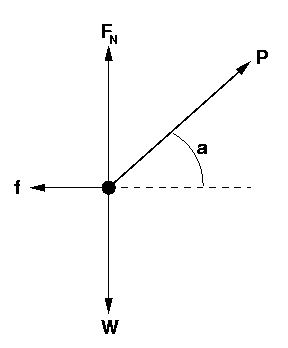# Forces on a crate with friction: find the normal force

• Jujubee37
And yes I understand the purpose of the problem. In summary, the conversation is about solving a physics problem involving a normal force and a vertical net force of zero. The conversation includes a discussion about the purpose of the problem and a clarification on plugging in the correct values for force P and kinetic frictional force f. The final answer is 104.79 N.f

#### Jujubee37

Homework Statement
Force P (78.28 N) pulls on a crate of weight W (164 N) on a rough surface at a constant speed. The figure shows the directions of the forces that act on the crate. FN represents the normal force. The kinetic frictional force is f (51.25 N). The angle, a, is 49.1 degrees. Calculate the value of the normal force.
Relevant Equations
mg-Fsin(x)I have attempted this problem by solving for the normal force. (16.73)(9.8)-51.25(sin)(49.1). I tried to work that out but it was incorrect because apparently the vertical force is zero. Could I get an explanation on what that means and where I should start?

I did. (16.73)(9.8)-51.25(sin)(49.1)= 125.21 N

Are these even in the same direction ?

Are these even in the same direction ?
I wouldn't know. All I know is that the vertical net force is zero and the normal force is one of the vertical forces. I worked this out two ways. one with sin and the other with cos because those are the only equations that I have involving an angle.

I wouldn't know.
Huh ? Isn't mg vertical and 51.25 N horizontal ?

apparently the vertical force is zero
Says who ?

Huh ? Isn't mg vertical and 51.25 N horizontal ?

Says who ?
Lon capa the website. I don't understand this so I can't answer your questions.

I can't get in there, so you have to play go-between.

Is it clar to you that ##\ mg\ ##, ##\ \ P\sin a\ \ ## and ## \ N \ ## are the only vertical forces in the story ?
And if ##N## would be zero, then ##\ mg = P\sin a\ \ ## would follow ?

Just to humor me, calculate the net horizontal force ...

##\ ##

I can't get in there, so you have to play go-between.

Is it clar to you that ##\ mg\ ##, ##\ \ P\sin a\ \ ## and ## \ N \ ## are the only vertical forces in the story ?
And if ##N## would be zero, then ##\ mg = P\sin a\ \ ## would follow ?

Just to humor me, calculate the net horizontal force ...

##\ ##
I still do not understand what I have to do. Solve for the horizontal? or what?

Why not just do it ?

##N## can't be zero: ##mg## is much bigger than ##P\sin a##.

Why not just do it ?

##N## can't be zero: ##mg## is much bigger than ##P\sin a##.
Do what? I really do not understand where I am supposed to start. I have no understanding of this problem. This is what the hint was for this problem.

 The net vertical force here is zero. The normal force is one of the vertical forces. Others are weight and part of the pulling force.

Aha ! It says the NET vertical force is zero. There is no acceleration in the vertical direction. So you have ##mg## down, ##P\sin a## and ##N## both up. And the sum is zero.

The only difference with your working is that you used 51.25 instead of 78.28 N for ##P##.

I did. (16.73)(9.8)-51.25(sin)(49.1)= 125.21 N
Where that 16.73 comes from?

I guess it's a calculated ##m##

•Lnewqban
Thank you, BvU; exactly.
Perhaps not being at scale, the posted diagram is confusing the OP.

However, I suspect @Jujubee37 is still confused ?

... I really do not understand where I am supposed to start. I have no understanding of this problem.

Not being pulled in any direction, the block pushes the ground down with force W of magnitude 164 N and there is no kinetic frictional force f to talk about.
Do you understand that?

Do you now understand the purpose of this problem?

@Jujubee37: You do not need to find the mass. Simply replace 'mg' by '164' (Newtons).

Have you solved the problem now, or do you still want help?

Homework Statement:: Force P (78.28 N) ... The kinetic frictional force is f (51.25 N).

View attachment 277913
. (16.73)(9.8)-51.25(sin)(49.1).
Looks to me that you got confused between P and f when plugging numbers into your equation.

•